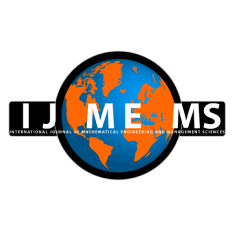### International Journal of Mathematical, Engineering and Management Sciences

#### ISSN: 2455-7749 . Open Access

Image Cryptography Based On A Second-Order QRT Difference Equation

#### Image Cryptography Based On A Second-Order QRT Difference Equation

Agus Sutrisno
Department of Mathematics, Faculty of Mathematics and Natural Science, Universitas Lampung, Bandar Lampung, Indonesia.

Aang Nuryaman
Department of Mathematics, Faculty of Mathematics and Natural Science, Universitas Lampung, Bandar Lampung, Indonesia.

Muslim Ansori
Department of Mathematics, Faculty of Mathematics and Natural Science, Universitas Lampung, Bandar Lampung, Indonesia.

Department of Mathematics, Faculty of Mathematics and Natural Science, Universitas Lampung, Bandar Lampung, Indonesia.

;
Accepted on February 02, 2022

Abstract

The use of mathematical concepts of mapping in cryptography provides advantages in securing text or image data. The qualitative properties of mapping can preserve data that is kept confidential. Two of the essential properties in the mapping are the reversible and the preserving area. In this article, besides constructing a linear mapping derived from a second-order QRT difference equation and examining its qualitative properties, the coding procedure is used to encrypt text and fractal images based on the two-dimensional linear maps. For the digital text and image security algorithms, we developed the pseudo code algorithm implemented in Mathematica®. The proposed encoding technique will be compared with a 2D mKdV linear map to demonstrate its efficacy.

Keywords- Fractal image, Cryptography, 2D QRT linear map, 2D mKdV linear map

Citation

Sutrisno, A., Nuryaman, A., Ansori, M., & Faisol, A. (2022). Image Cryptography Based On A Second-Order QRT Difference Equation. International Journal of Mathematical, Engineering and Management Sciences, 7(3), 399-416. https://doi.org/10.33889/IJMEMS.2022.7.3.027.